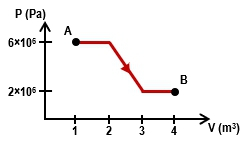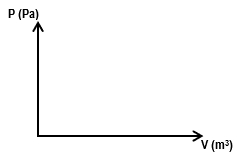Start typing, then use the up and down arrows to select an option from the list.## Physics

Learn the toughest concepts covered in Physics with step-by-step video tutorials and practice problems by world-class tutors

22. The First Law of Thermodynamics

# Work Done Through Multiple Processes

1
concept

## Calculating Works For Multiple Thermodynamic Processes7m
Play a video:
2
Problem

How much work is done on a gas that expands from A to B along the path shown below?3
Problem

A gas with an initial volume of 0.2 m3 is heated at constant volume, and the pressure increases from 2×105 Pa to 5×105. Then, it compresses at constant pressure until it reaches a final volume of 0.12 m3. Draw the two processes in the PV diagram below and find the total work done by the gas.